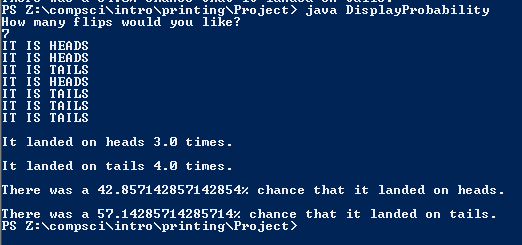PROGRAMMING AND CODE

# FINAL

## Code

```/// Name: Justin Li
/// Period 7
/// Program Name: DisplayProbability
/// File Name: DisplayProbability.java
/// Date Finished: 1/21/16

import java.util.Scanner;
import java.util.Random;

class DisplayProbability {

public static void main(String[]args) {

Random r = new Random();
Scanner keyboard = new Scanner(System.in);
int total, coin;
double probability, probability2, amount, heads, tails;
coin = r.nextInt(2);
tails = 0;
amount = 0;

do {
System.out.println("How many flips would you like?");
total = keyboard.nextInt();
} while ( total > 2100000000 || total < 1);
//I used a do while loop because it worked. lol
do {
if (coin == 1){
total = total - 1;
coin = r.nextInt(2);
amount = amount + 1;
}
else if (coin == 0) {
System.out.println("IT IS TAILS");
total = total - 1;
coin = r.nextInt(2);
tails = tails + 1;
amount = amount + 1;
}

} while ( total < 2100000000 && total > 0);

probability = heads / amount * 100;
probability2 = tails / amount * 100;